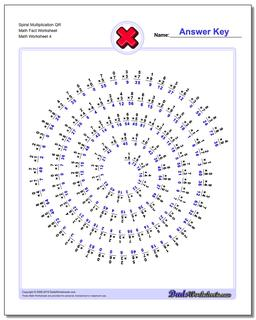PLEASE GO BACK AND USE THE BIG BLUE 'PRINT' BUTTON ON THE PAGE TO PRINT THE WORKSHEET CORRECTLY!Sorry for the trouble! The browser won't print the embedded worksheet PDF directly using the normal 'Print' command in the file menu, so you need to click the big 'Print' button to send just the worksheet and not the surrounding page to the printer.

# Math Worksheets: Multiplication: Multiplication: Spiral Multiplication QR Math Fact Worksheet (Fourth Worksheet)## Spiral Multiplication QR Math Fact Worksheet (Fourth Worksheet)

Property Value
DescriptionSpiral Multiplication QR Math Fact Worksheet: Tired of the same old math fact worksheets with rows and rows of problems? These multiplication worksheets present the facts in a spiral layout that provides fun a twist on memorizing the times tables. They use the same fact layouts as the spaceship math sheets above, so try the first two sets of worksheets if you are looking for all of the multiplication facts or for practice without the easier problems, or try the others in series for an incremental approach to learning the facts. (Fourth Worksheet)
Resource TypeWorksheet